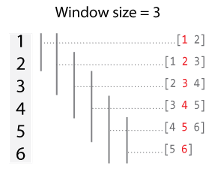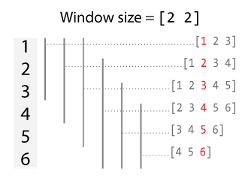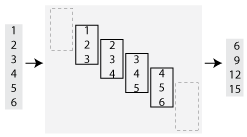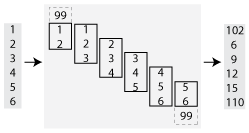# matlab.tall.blockMovingWindow

Apply moving window function and block reduction to padded blocks of data

## Syntax

``tA = matlab.tall.blockMovingWindow(windowfcn,blockfcn,window,tX)``
``[tA,tB,...] = matlab.tall.blockMovingWindow(windowfcn,blockfcn,window,tX,tY,...)``
``[___] = matlab.tall.blockMovingWindow(___,Name,Value)``

## Description

example

````tA = matlab.tall.blockMovingWindow(windowfcn,blockfcn,window,tX)` applies `blockfcn` to complete windows of data and `windowfcn` to incomplete windows of data near the edges. `window` specifies the size of the sliding window. The result contains the vertical concatenation of applying `blockfcn` and `windowfcn` to these windows of data.```

example

````[tA,tB,...] = matlab.tall.blockMovingWindow(windowfcn,blockfcn,window,tX,tY,...)`, where `windowfcn` and `blockfcn` are function handles that return multiple outputs, returns arrays `tA, tB, ...`, each corresponding to one of the output arguments of `windowfcn` and `blockfcn`. The inputs to `windowfcn` and `blockfcn` are pieces of data from the arguments ```tX, tY, ...```. This syntax has these requirements: `windowfcn` and `blockfcn` must return the same number of outputs as were requested from `matlab.tall.blockMovingWindow`.Each output of `windowfcn` and `blockfcn` must be the same type as the first data input `tX`.All outputs `tA,tB,...` must have the same height. ```

example

````[___] = matlab.tall.blockMovingWindow(___,Name,Value)` specifies additional options with one or more name-value pair arguments using any of the previous syntaxes. For example, to adjust the step size between windows, you can specify `'Stride'` and a scalar. Or to change the treatment of endpoints where there are not enough elements to complete a window, you can specify `'EndPoints'` and a valid option (`'shrink'`, `'discard'`, or a numeric padding value).```

## Examples

collapse all

Use `matlab.tall.blockMovingWindow` to calculate the moving mean of airline arrival and departure delays.

Create a datastore for the `airlinesmall.csv` data set and convert it into a tall array. The data contains information about arrival and departure times of US flights. Extract the `ArrDelay` and `DepDelay` variables, which are vectors of flight delays, to create a tall array containing the delays as separate columns.

```varnames = {'ArrDelay', 'DepDelay'}; ds = tabularTextDatastore('airlinesmall.csv', 'TreatAsMissing', 'NA', ... 'SelectedVariableNames', varnames); tt = tall(ds); tX = [tt.ArrDelay tt.DepDelay]```
```tX = Mx2 tall double matrix 8 12 8 1 21 20 13 12 4 -1 59 63 3 -2 11 -1 : : : : ```

Use `matlab.tall.blockMovingWindow` to calculate the moving mean of the data in the first dimension with a window size of 10. Since `windowfcn` applies only to single windows of data, you can use the `mean` function to reduce the windows of data down into a matrix with one row. The `blockfcn` applies to whole blocks of data, so use the `movmean` function to calculate the mean of each full window of data in the blocks.

```windowfcn = @(info,x) mean(x,1,'omitnan'); blockfcn = @(info,x) movmean(x,info.Window,1,'omitnan','EndPoints','discard'); A = matlab.tall.blockMovingWindow(windowfcn, blockfcn, 10, tX)```
```A = MxNx... tall double array ? ? ? ... ? ? ? ... ? ? ? ... : : : : : : ```

Gather a portion of the results into memory.

`gather(A(1:10,:))`
```Evaluating tall expression using the Local MATLAB Session: - Pass 1 of 2: Completed in 0.96 sec - Pass 2 of 2: Completed in 5 sec Evaluation completed in 6.7 sec ```
```ans = 10×2 10.8000 8.8000 18.8333 17.8333 16.5714 15.0000 15.8750 13.0000 14.4444 11.8889 13.2000 10.8000 14.0000 11.1000 13.5000 11.9000 15.3000 11.4000 19.7000 13.4000 ```

Calculate moving statistics on the variables of a table.

Load the `outages.csv` data set as a tall table. The data contains information about power outages.

`T = tall(readtable('outages.csv'))`
```T = 1,468x6 tall table Region OutageTime Loss Customers RestorationTime Cause _____________ ________________ ______ __________ ________________ ___________________ {'SouthWest'} 2002-02-01 12:18 458.98 1.8202e+06 2002-02-07 16:50 {'winter storm' } {'SouthEast'} 2003-01-23 00:49 530.14 2.1204e+05 NaT {'winter storm' } {'SouthEast'} 2003-02-07 21:15 289.4 1.4294e+05 2003-02-17 08:14 {'winter storm' } {'West' } 2004-04-06 05:44 434.81 3.4037e+05 2004-04-06 06:10 {'equipment fault'} {'MidWest' } 2002-03-16 06:18 186.44 2.1275e+05 2002-03-18 23:23 {'severe storm' } {'West' } 2003-06-18 02:49 0 0 2003-06-18 10:54 {'attack' } {'West' } 2004-06-20 14:39 231.29 NaN 2004-06-20 19:16 {'equipment fault'} {'West' } 2002-06-06 19:28 311.86 NaN 2002-06-07 00:51 {'equipment fault'} : : : : : : : : : : : : ```

Use `matlab.tall.blockMovingWindow` to apply a moving-window function to blocks of the tall table. Specify these options:

• `blkstats` as the block function to operate on complete blocks of data (included at the end of the example as a local function).

• A window size of 50 and a stride of 5.

• `EndPoints` as `'discard'` to ignore incomplete windows of data. With this value, the `windowfcn` input can be specified as empty `[]` since only complete windows of data are operated on.

• The input table has six variables, but the two outputs are double-precision vectors. Specify scalar doubles as the value for `OutputsLike` so that the function permits this change in data type and size.

```[A, B] = matlab.tall.blockMovingWindow([], @blkstats, 50, T, 'Stride', 5, ... 'EndPoints', 'discard', 'OutputsLike', {1, 1});```

Preview a few rows in the results.

`[A,B] = gather(head(A),head(B))`
```Evaluating tall expression using the Local MATLAB Session: - Pass 1 of 2: Completed in 0.42 sec - Pass 2 of 2: Completed in 0.64 sec Evaluation completed in 1.8 sec ```
```A = 8×1 254.0861 254.0861 340.3499 452.0191 464.8524 471.9737 464.8524 464.8524 ```
```B = 8×1 105 × 1.3447 1.0779 1.4227 1.4509 1.2888 1.2888 1.2308 1.3722 ```

The `blkstats` function calculates the moving median value of the `Loss` and `Customers` table variables in the first dimension using the specified window size. The function applies the `Stride` value to reduce the size of the output, and then it returns the results as two vectors.

```function [out1, out2] = blkstats(info, t) a = movmedian([t.Loss t.Customers], info.Window, 1, 'omitnan', 'EndPoints', 'discard'); a = a(1:info.Stride:end, :); out1 = a(:,1); out2 = a(:,2); end```

## Input Arguments

collapse all

Function to apply to incomplete windows of data, specified as a function handle, anonymous function, or `[]`. `windowfcn` is invoked once per incomplete window as the calculation moves over data in the tall dimension. `matlab.tall.blockMovingWindow` applies `windowfcn` only when `'EndPoints'` has the default value of `'shrink'`. If you specify a different value for `'EndPoints'`, then set `windowfcn` to `[]`.

Each output of `windowfcn` must be the same type as the first data input `tX`. You can use the `'OutputsLike'` option to return outputs of different data types.

The general functional signature of `windowfcn` is

`[a, b, c, ...] = windowfcn(info, x, y, ...)`
The `info` input is a structure provided by `matlab.tall.blockMovingWindow` that includes these fields:

• `Stride` — Specified step size between windows (default: 1). Set this value with the `'Stride'` name-value pair.

• `Window` — Specified window size. Set this value with the `window` input argument.

`windowfcn` must satisfy these requirements:

1. Input Arguments — The inputs ```[x, y, z, ...]``` are blocks of data that fit in memory. The blocks are produced by extracting data from the respective tall array inputs ```[tX, tY, tZ, ...]```. The inputs `[x, y, z, ...]` satisfy these properties:

• All of the inputs `[x, y, z, ...]` have the same size in the first dimension.

• The blocks of data in `[x, y, z, ...]` come from the same index in the tall dimension, assuming the tall array is nonsingleton in the tall dimension. For example, if `tX` and `tY` are nonsingleton in the tall dimension, then the first set of blocks might be `x = tX(1:20000,:)` and `y = tY(1:20000,:)`.

• When the first dimension of any of `[tX, tY, tZ, ...]` has a size of `1`, the corresponding block ```[x, y, z, ...]``` consists of all the data in that tall array.

• Applying `windowfcn` must result in a reduction of the input data to a scalar or a slice of an array of height 1.

When the input is a matrix, N-D array, table, or timetable, applying `windowfcn` must result in a reduction of the input data in each of its columns or variables.

2. Output Arguments — The outputs ```[a, b, c, ...]``` are blocks that fit in memory to be sent to the respective outputs `[tA, tB, tC, ...]`. The outputs ```[a, b, c, ...]``` satisfy these properties:

• All of the outputs `[a, b, c, ...]` must have the same size in the first dimension.

• All of the outputs `[a, b, c, ...]` are vertically concatenated with the respective results of previous calls to `windowfcn`.

• All of the outputs `[a, b, c, ...]` are sent to the same index in the first dimension in their respective destination output arrays.

3. Functional Rules`windowfcn` must satisfy this functional rule:

• `F([inputs1; inputs2]) == [F(inputs1); F(inputs2)]`: Applying the function to the concatenation of the inputs should be the same as applying the function to the inputs separately and then concatenating the results.

Example: ```A = matlab.tall.blockMovingWindow(@windowfcn, @blockfcn, 10, tX)```

Example: ```A = matlab.tall.blockMovingWindow([], @blockfcn, 10, tX, 'EndPoints', 'discard')```

Data Types: `function_handle`

Function to apply to blocks of data, specified as a function handle or anonymous function. `blockfcn` is applied to blocks of data that contain complete windows of data. Thus, `blockfcn` must operate in a vectorized manner on entire blocks of data and return output that has the proper size for the specified window size and stride.

Each output of `blockfcn` must be the same type as the first data input `tX`. You can use the `'OutputsLike'` option to return outputs of different data types.

`matlab.tall.blockMovingWindow` applies `blockfcn` to blocks of data whenever the block contains only complete windows:

• For middle blocks when `'EndPoints'` is set to `'shrink'` (default behavior). In this case `windowfcn` operates on the incomplete windows of data on the ends.

• For all blocks when `'EndPoints'` is set to `'discard'` or a padding value.

The general functional signature of `blockfcn` is

`[a, b, c, ...] = blockfcn(info, bX, bY, bZ, ...)`
The `info` input is a structure provided by `matlab.tall.blockMovingWindow` that includes these fields:

• `Stride` — Specified step size between windows (default: 1). Set this value with the `'Stride'` name-value pair.

• `Window` — Specified window size. Set this value with the `window` input argument.

The blocks of data `bX, bY, bZ, ...` that `matlab.tall.blockMovingWindow` provides to `blockfcn` have these properties:

• The blocks contain only full-sized windows. `blockfcn` does not have to define a behavior for incomplete windows of data.

• The first window of data starts at the first element of the block. The last element of the last window is the last element of the block.

`blockfcn` must satisfy these requirements:

1. Input Arguments — The inputs ```[bX, bY, bZ, ...]``` are blocks of data that fit in memory. The blocks are produced by extracting data from the respective tall array inputs ```[tX, tY, tZ, ...]```. The inputs `[bX, bY, bZ, ...]` satisfy these properties:

• All of the inputs `[bX, bY, bZ, ...]` have the same size in the first dimension after any allowed expansion.

• The blocks of data in `[bX, bY, bZ, ...]` come from the same index in the tall dimension, assuming the tall array is nonsingleton in the tall dimension. For example, if `tX` and `tY` are nonsingleton in the tall dimension, then the first set of blocks might be `bX = tX(1:20000,:)` and `bY = tY(1:20000,:)`.

• If the first dimension of any of the data inputs ```[tX, tY, tZ, ...]``` has a size of `1`, then the corresponding block `[bX, bY, bZ, ...]` consists of all the data in that tall array.

• Applying `blockfcn` must result in a reduction of the input data such that the result has height equal to the number of windows in the block. You can use `info.Window` and `info.Stride` to determine the number of windows in a block.

If the input is a matrix, N-D array, table, or timetable, then applying `blockfcn` must result in a reduction of the input data in each of its columns or variables.

2. Output Arguments — The outputs ```[a, b, c, ...]``` are blocks that fit in memory, to be sent to the respective outputs `[tA, tB, tC, ...]`. The outputs ```[a, b, c, ...]``` satisfy these properties:

• All of the outputs `[a, b, c, ...]` must have the same size in the first dimension.

• All of the outputs `[a, b, c, ...]` are vertically concatenated with the respective results of previous calls to `blockfcn`.

• All of the outputs `[a, b, c, ...]` are sent to the same index in the first dimension in their respective destination output arrays.

3. Functional Rules`blockfcn` must satisfy this functional rule:

• `F([inputs1; inputs2]) == [F(inputs1); F(inputs2)]`: Applying the function to the concatenation of the inputs should be the same as applying the function to the inputs separately and then concatenating the results.

Example: ```A = matlab.tall.blockMovingWindow(@windowfcn, @blockfcn, 10, tX)```

Example: ```A = matlab.tall.blockMovingWindow([], @blockfcn, 10, tX, 'EndPoints', 'discard')```

Data Types: `function_handle`

Window size, specified as a positive integer scalar or a two-element row vector `[NB NF]`.

• If `window` is a scalar, then:

• When the window size is odd, each window is centered on the corresponding element in the data.• When the window size is even, each window is centered about the current and previous elements.• If `window` is a vector `[NB NF]`, then the window includes the previous `NB` elements, the current element, and the next `NF` elements of the inputs.By default, the window size is automatically truncated at the endpoints when not enough elements are available to fill the window. When the window is truncated in this manner, the function operates only on the elements that fill the window. You can change this behavior with the `EndPoints` name-value pair.

Data Types: `single` | `double` | `int8` | `int16` | `int32` | `int64` | `uint8` | `uint16` | `uint32` | `uint64`

Input arrays, specified as separate arguments of scalars, vectors, matrices, multidimensional arrays, tables, or timetables. The input arrays can be tall or in-memory arrays. The input arrays are used as inputs to the transform function `fcn`. Each input array `tX,tY,...` must have the same height.

### Name-Value Arguments

Specify optional pairs of arguments as `Name1=Value1,...,NameN=ValueN`, where `Name` is the argument name and `Value` is the corresponding value. Name-value arguments must appear after other arguments, but the order of the pairs does not matter.

Before R2021a, use commas to separate each name and value, and enclose `Name` in quotes.

Example: ```tA = matlab.tall.blockMovingWindow(@windowfcn, blockfcn, window, tX, 'Stride', 2)```

Step size between windows, specified as the comma-separated pair consisting of `'Stride'` and a positive integer scalar. After `fcn` operates on a window of data, the calculation advances by the `'Stride'` value before operating on the next window. Increasing the value of `'Stride'` from the default value of 1 is the same as reducing the size of the output by picking out every other element, or every third element, and so on.

By default, the value of `'Stride'` is `1`, so that each window is centered on each element in the input. For example, here is a moving sum calculation with a window size of 3 operating on the vector `[1 2 3 4 5 6]'`:If the value of `'Stride'` is `2`, then the calculation changes so that each window is centered on every second element in the input (1, 3, 5). The moving sum now returns three partial sums rather than six:Data Types: `single` | `double` | `int8` | `int16` | `int32` | `int64` | `uint8` | `uint16` | `uint32` | `uint64`

Method to treat leading and trailing windows, specified as the comma-separated pair consisting of `'EndPoints'` and one of the values in the table.

At the beginning and end of a windowed calculation, the window of elements being operated on is incomplete. The `'EndPoints'` option specifies how to treat these incomplete windows.

`'EndPoints'` ValueDescriptionExample: Moving Sum

`'shrink'`

Shrink the window size near the endpoints of the input to include only existing elements.`'discard'`

Do not output any results where the window does not completely overlap with existing elements.Substitute nonexisting elements with a specified numeric or logical value.

• The padding value must have the same type as `tX`.

• The size of the padding value in the first dimension must be equal to 1, and the size in other dimensions must match `tX`.Data Types: `single` | `double` | `int8` | `int16` | `int32` | `int64` | `uint8` | `uint16` | `uint32` | `uint64` | `logical` | `char` | `string`

Prototype of output arrays, specified as the comma-separated pair consisting of `'OutputsLike'` and a cell array containing prototype arrays. When you specify `'OutputsLike'`, the output arrays `tA,tB,...` returned by `matlab.tall.blockMovingWindow` have the same data types and attributes as the specified prototype arrays `{PA,PB,...}`. You must specify `'OutputsLike'` whenever the data type of an output array is different than that of the input array. If you specify `'OutputsLike'`, then you must specify a prototype array for each output.

Example: `tA = matlab.tall.blockMovingWindow(..., tX, 'OutputsLike', {int8(1)});`, where `tX` is a double-precision tall array, returns `tA` as `int8` instead of `double`.

Data Types: `cell`

## Output Arguments

collapse all

Output arrays, returned as scalars, vectors, matrices, or multidimensional arrays. If any input to `matlab.tall.blockMovingWindow` is tall, then all output arguments are also tall. Otherwise, all output arguments are in-memory arrays.

• The size and data type of the output arrays depend on the specified window functions `windowfcn` and `blockfcn`.

• The output arrays `tA,tB,...` all have the same height, which depends on the value of `'Stride'` and `'EndPoints'`. By default the output arrays are the same size as the input arrays.

• In general, the outputs `tA,tB,...` must all have the same data type as the first data input `tX`. However, you can specify `'OutputsLike'` to return different data types. In cases where the input arrays `tX, tY, ...` are empty, or when `'EndPoints'` is `'discard'` and there are not enough elements to fill a full-sized window, `matlab.tall.blockMovingWindow` returns empty outputs. The sizes of the empty outputs are based on the size of the input array `tX`, or on the sizes of the prototype arrays provided to `'OutputsLike'`, if specified.

## Tips

• Use `matlab.tall.movingWindow` for simple sliding-window calculations. `matlab.tall.blockMovingWindow` is an advanced API designed to provide more flexibility to perform sliding-window calculations on tall arrays. As such, it is more complicated to use since the functions must accurately process blocks of data that contain many complete windows. However, with properly vectorized calculations, you can reduce the necessary number of function calls and improve performance.

## Version History

Introduced in R2019a